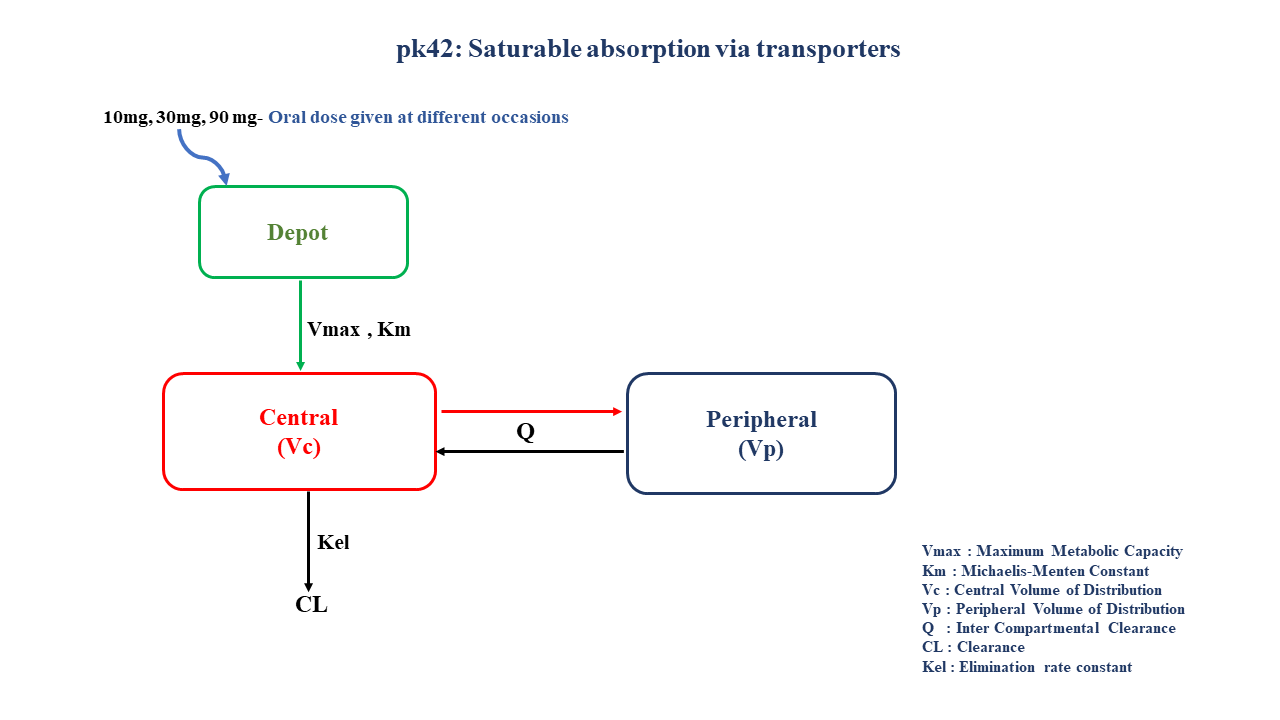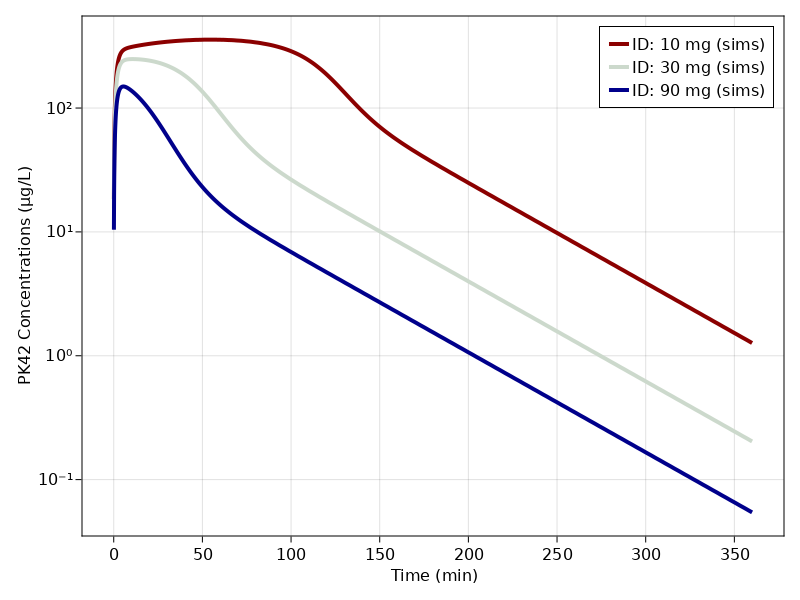# Exercise PK42 - Saturable absorption via transporters

### Background

• Structural model - Multi compartment model with saturable absorption kinetics

• Route of administration - Oral Route

• Dosage Regimen - 10 mg, 30 mg, 90 mg oral dose given at different occasions

• Number of Subjects - 1### Learning Outcomes

This model gives an understanding of non linear absorption due to saturation of drug transporters at higher doses of drug administered orally.

### Objectives

In this tutorial, you will learn to build a multi compartment model for a drug following saturable absorption kinetics and simulate the data.

### Libraries

Call the "necessary" libraries to get started.

using Random
using Pumas
using PumasUtilities
using CairoMakie


### Model

In this multi compartment model,a single subject receives oral doses of a compound X at three different occasions which follows non linear absorption and linear disposition kinetics.

pk_42           = @model begin
desc        = "Non-linear Absorption Model"
timeu       = u"minute"
end

@param begin
"Maximum Metabolic Capacity (ug/min)"
tvvmax      ∈ RealDomain(lower=0)
"Michaelis-Menten Constant (ug/L)"
tvkm        ∈ RealDomain(lower=0)
"Volume of Distribution of Central Compartment (L)"
tvvc        ∈ RealDomain(lower=0)
"Volume of Distribution of Peripheral compartment (L)"
tvvp        ∈ RealDomain(lower=0)
"Inter Compartmental Clearance (L/min)"
tvq         ∈ RealDomain(lower=0)
"Clearance (L/min)"
tvcl        ∈ RealDomain(lower=0)
Ω           ∈ PDiagDomain(6)
end

@random begin
η           ~ MvNormal(Ω)
end

@pre begin
Vmax        = tvvmax*exp(η)
Km          = tvkm*exp(η)
Vc          = tvvc*exp(η)
Vp          = tvvp*exp(η)
Q           = tvq*exp(η)
CL          = tvcl*exp(η)
end

@vars begin
VMKM       := Vmax/(Km+Depot)
end

@dynamics begin
Depot'      = -VMKM*Depot
Central'    =  VMKM*Depot -CL*(Central/Vc) - (Q/Vc)*Central + (Q/Vp)*Peripheral
Peripheral' = (Q/Vc)*Central - (Q/Vp)*Peripheral
end

@derived begin
cp          = @. Central/Vc
"""
Observed Concentration (ug/L)
"""
end
end

PumasModel
Parameters: tvvmax, tvkm, tvvc, tvvp, tvq, tvcl, Ω, σ_add
Random effects: η
Covariates:
Dynamical variables: Depot, Central, Peripheral
Derived: cp, dv
Observed: cp, dv


### Parameters

Parameters provided for simulation as below. tv represents the typical value for parameters.

• $Vmax$ - Maximum Metabolic Capacity (ug/min)

• $Km$ - Michaelis-Menten Constant (ug/ml)

• $Vc$ - Volume of Distribution of Central Compartment (L)

• $Vp$ - Volume of Distribution of Peripheral compartment (L)

• $Q$ - Inter Compartmental Clearance (L/min)

• $CL$ - Clearance (L/min)

• $Ω$ - Between Subject Variability

• $σ$ - Residual Error

param = (tvvmax = 982.453,
tvkm   = 9570.63,
tvvc   = 4.66257,
tvvp   = 35,
tvq    = 0.985,
tvcl   = 2.00525,
Ω      = Diagonal([0.0, 0.0, 0.0,0.0,0.0,0.0]),

(tvvmax = 982.453, tvkm = 9570.63, tvvc = 4.66257, tvvp = 35, tvq = 0.985,
tvcl = 2.00525, Ω = [0.0 0.0 … 0.0 0.0; 0.0 0.0 … 0.0 0.0; … ; 0.0 0.0 … 0.
0 0.0; 0.0 0.0 … 0.0 0.0], σ_add = 0.123)


### Dosage Regimen

A single subject received oral dosing of 10,30,90 mg on three different occasions

dose_ind = [90000,30000,10000]
ids = ["10 mg","30 mg", "90 mg"]
ev(x) = DosageRegimen(dose_ind[x], time=0, cmt=1)
pop     = map(i -> Subject(id=ids[i], events=ev(i)), 1:length(ids))

Population
Subjects: 3
Observations:


### Simulation

Simulate the plasma concentration for single subject after an oral dose given at three different occasions.

Random.seed!(123)
sim_pop3_sub = simobs(pk_42, pop, param, obstimes=0.1:0.1:360)


### Visualization

f1, a1, p1 = sim_plot(pk_42, sim_pop3_sub,
observations = :cp,
color = :redsblues,
linewidth = 4,
axis = (
xlabel = "Time (min)",
ylabel = "PK42 Concentrations (μg/L)",
xticks = 0:50:400, yscale = log10))
axislegend(a1)
f1